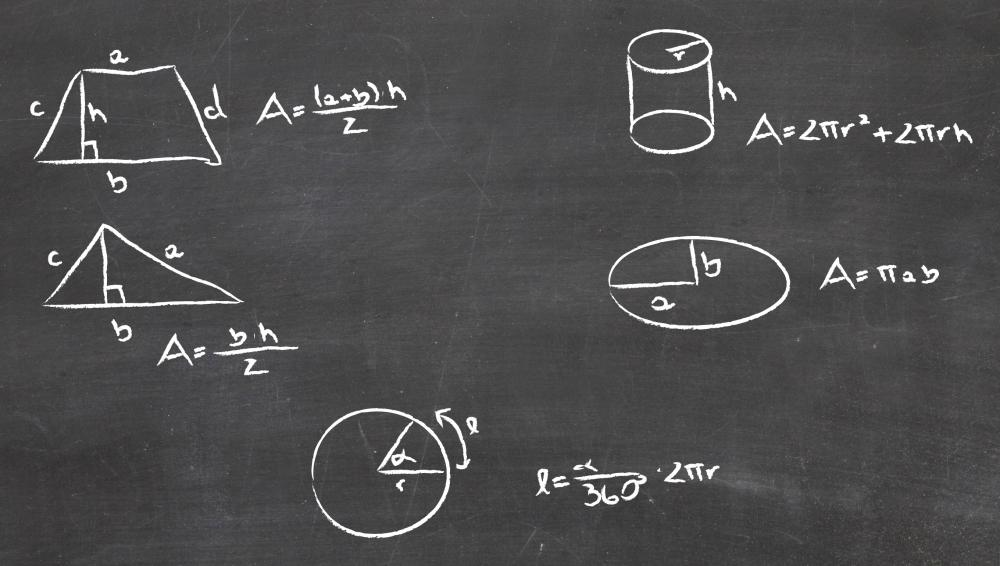# What is the Pythagorean Theorem?Mary McMahonMary McMahonThe Pythagorean theorem was used to make complex engineering calculations for the Space Shuttle.

The Pythagorean theorem is a mathematical theorem named after Pythagoras, a Greek mathematician who lived around the fifth century BCE. Pythagoras is usually given the credit for coming up with the theorem and providing early proofs, although evidence suggests that the theorem actually predates the existence of Pythagoras, and that he may simply have popularized it. Whoever deserves the credit for developing the Pythagorean theorem would undoubtedly be pleased to know that it is taught in geometry classes all over the world, and it is utilized on a daily basis for everything from doing high school math homework to making complex engineering calculations for the Space Shuttle.The Pythagorean theorem is used to calculate the sides of a triangle.

According to the Pythagorean theorem, if the lengths of the sides of a right triangle are squared, the sum of the squares will equal the length of the hypotenuse squared. This theorem is often expressed as a simple formula: a²+b²=c², with a and b representing the sides of the triangle, while c represents the hypotenuse. In a simple example of how this theorem might be used, someone might be wondering about how long it would take to cut across a rectangular lot of land, rather than skirting the edges, relying on the principle that a rectangle can be divided into two simple right triangles. He or she could measure two adjoining sides, determine their squares, add the squares together, and find the square root of the sum to determine the length of the lot's diagonal.

Like other mathematical theorems, the Pythagorean theorem relies on proofs. Each proof is designed to create more supporting evidence to show that the theorem is correct, by demonstrating various applications, showing the shapes that the Pythagorean theorem cannot be applied to, and attempting to disprove the theorem to show, in reverse, that the logic behind the theorem is sound. Because the Pythagorean theorem is one of the oldest math theorems in use today, it is also one of the most heavily proved, with hundreds of proofs by mathematicians throughout history adding to the body of evidence which shows that the theorem is valid.

Some special shapes can be described with the Pythagorean theorem. A Pythagorean triple is a right triangle in which the lengths of the sides and hypotenuse are all whole numbers. The smallest Pythagorean triple is a triangle in which a=3, b=4, and c=5. Using the Pythagorean theorem, people can see that 9+16=25. The squares in the theorem can also be literal; if one were to use every length of a right triangle as the side of a square, the squares of the sides would have the same area as the square created by the length of the hypotenuse.

One can use this theorem to find the length of any unknown segment in a right triangle, making the formula useful for people who want to find the distance between two points. If, for example, one knows that one side of a right triangle is equal to three, and the hypotenuse is equal to five, one knows that the other side is four long, relying on the well-known Pythagorean triple discussed above.Mary McMahon

Ever since she began contributing to the site several years ago, Mary has embraced the exciting challenge of being a wiseGEEK researcher and writer. Mary has a liberal arts degree from Goddard College and spends her free time reading, cooking, and exploring the great outdoors.Mary McMahon

Ever since she began contributing to the site several years ago, Mary has embraced the exciting challenge of being a wiseGEEK researcher and writer. Mary has a liberal arts degree from Goddard College and spends her free time reading, cooking, and exploring the great outdoors.

## You might also Like## Discuss this Article

Forgot password?
###### Register:
•The Pythagorean theorem was used to make complex engineering calculations for the Space Shuttle.
•The Pythagorean theorem is used to calculate the sides of a triangle.